Chapter No. 3 – Vouchers and Transactions – Unimax Publications Class 11 – 2021

In this article, We have provided the Question wise solution of all Questions of Chapter No. 3 – Vouchers and Transactions – Unimax Publications Class 11 – 2021, Vouchers and Transaction are also known as Accouting Equation so first of all, we have explained the meaning of Accounting Equation: –

What is the Accounting Equation:

Accounting Equation is the basis of the double-entry system of accounting. The double-entry system means every business transaction has two aspects one is the Debit and another is the Credit. Example: – If we want to buy something like coke, pizza or burger we have to pay cash for these items, So in this transaction, we are receiving food and paying cash which means we are doing two jobs, that is why it is called double-entry system.

In the double-entry system, every Debit amount should be equal to the Credit amount Because :
The Business can receive cash or assets from two sources, one from an owner and another is a third party. When it received from an owner this is called Capital and when receiving from third parties then it is called liabilities.

So, in Accounting Equation, Cash or Assets always Equal to Capital and Liabilities of the Business.

“Assets = Liabilities + Capital“

What is Accounting Equation | Example

Check Meaning of Accounting Equation in Hindi from the following link:

What is the Accounting Equation- In Hindi

Chapter No. 3 – Vouchers and Transactions – Unimax Publications Class 11 – 2021 – Solution:

Question wise solution of all Questions of Chapter No. 3 – Vouchers and Transactions – Unimax Publications Class 11 – 2021 – Solution are shown below: –Question 1 Chapter 3 - Unimax 1. If the capital of a business is Rs. 1,20,000 and liabilities are Rs ...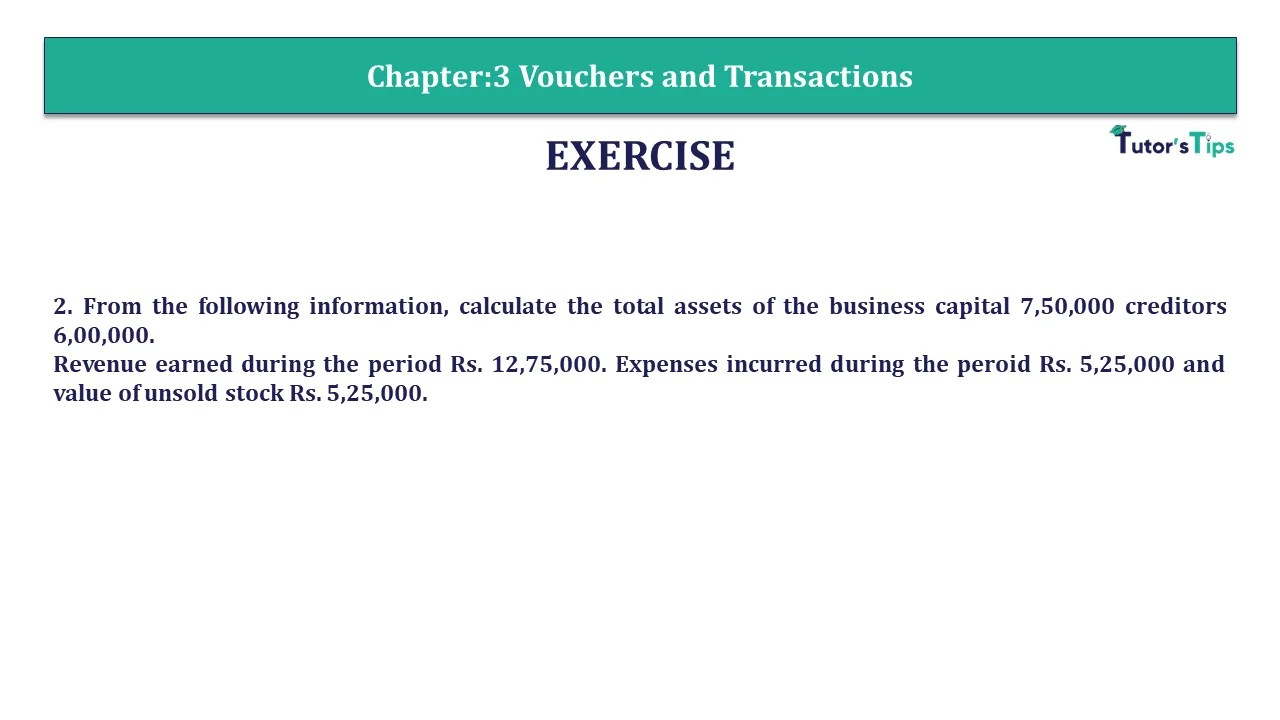Question 2 Chapter 3 - Unimax 2. From the following information, calculate the total assets of the business capital 7,50,000 ...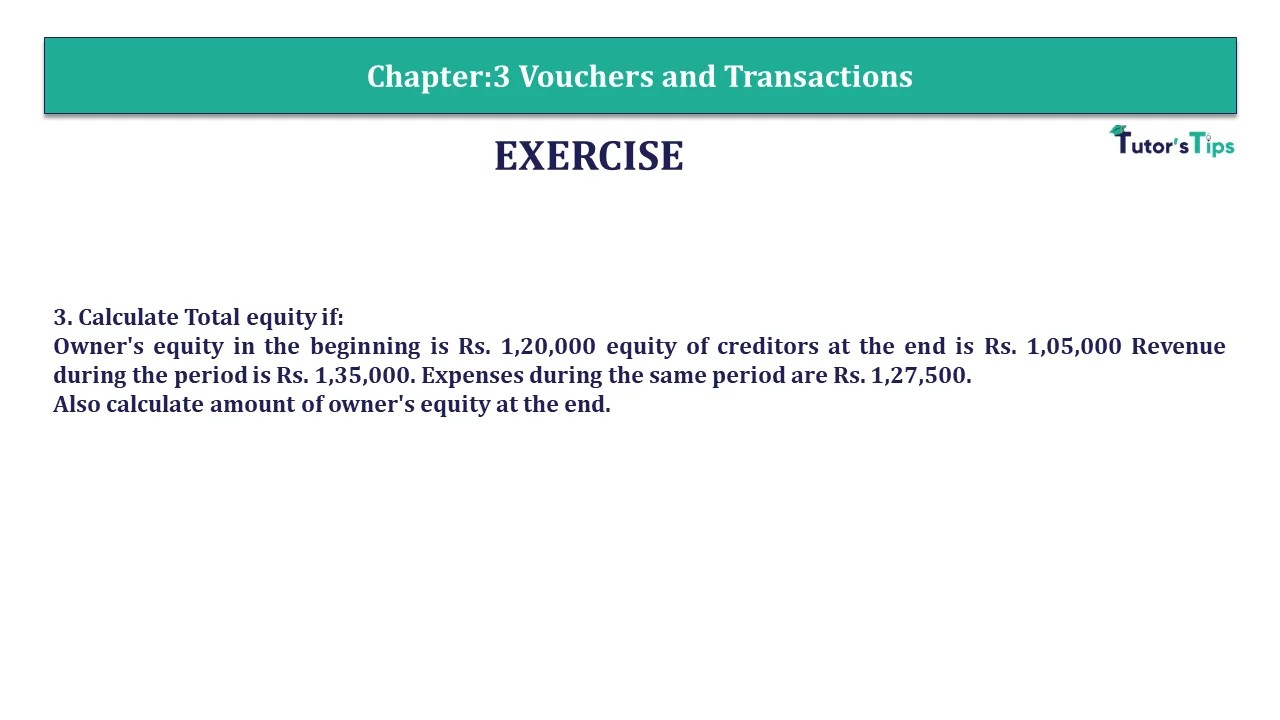Question 3 Chapter 3 - Unimax 3. Calculate Total equity if: Owner's equity in the beginning is Rs. 1,20,000 equity ...Question 4 Chapter 3 - Unimax 4. A commenced his cloth business on 1st April, 2020 with a capital of ...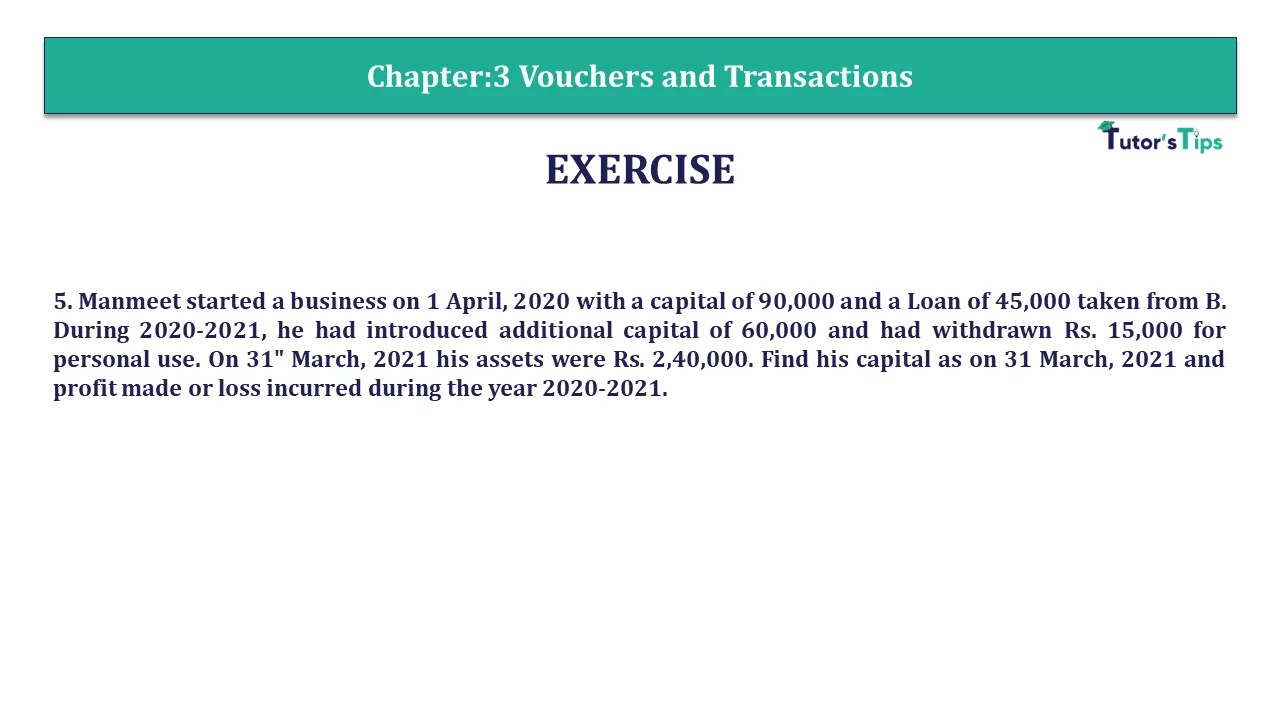Question 5 Chapter 3 - Unimax 5. Manmeet started a business on 1 April, 2020 with a capital of 90,000 ...Question 6 Chapter 3 - Unimax 6. On 31st March, 2021 the total assets and external Liabilities were Rs. 3,00,000 ...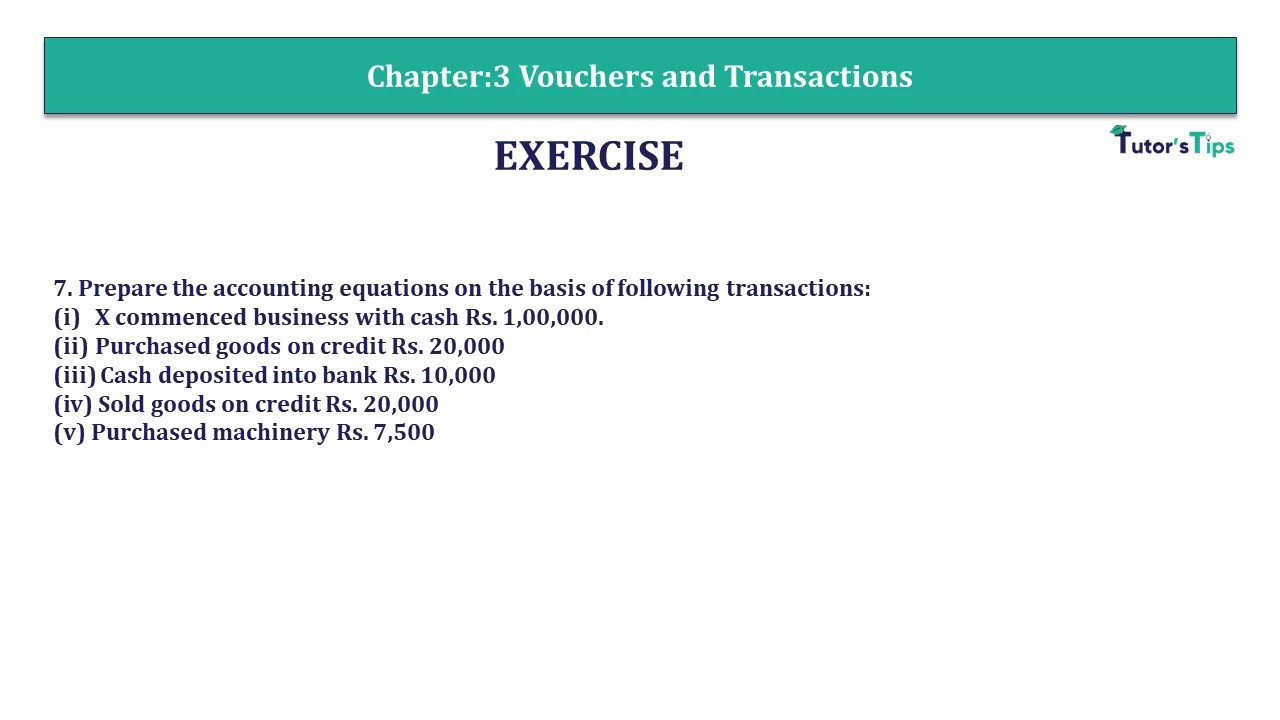Question 7 Chapter 3 - Unimax 7. Prepare the accounting equations on the basis of following transactions:(i) X commenced business ...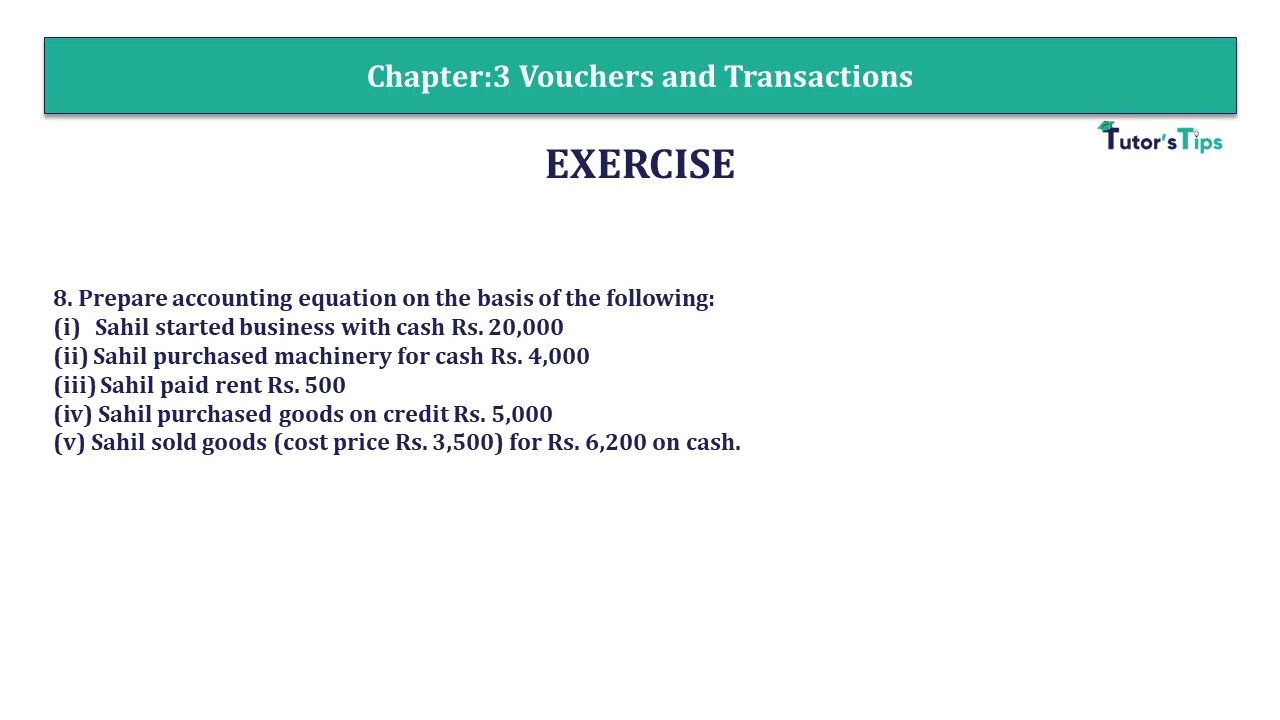Question 8 Chapter 3 - Unimax 8. Prepare accounting equation on the basis of the following: (i) Sahil started business ...Question 9 Chapter 3 - Unimax 9. Prepare Accounting equation on the basis of following: (i) Mandeep started business with ...Question 10 Chapter 3 - Unimax 10. Prove that the accounting equation is satisfied in all the following transactions Sandeep ...Question 11 Chapter 3 - Unimax 11. Prepare accounting equation on the basis of following transaction. (i) Started business with ...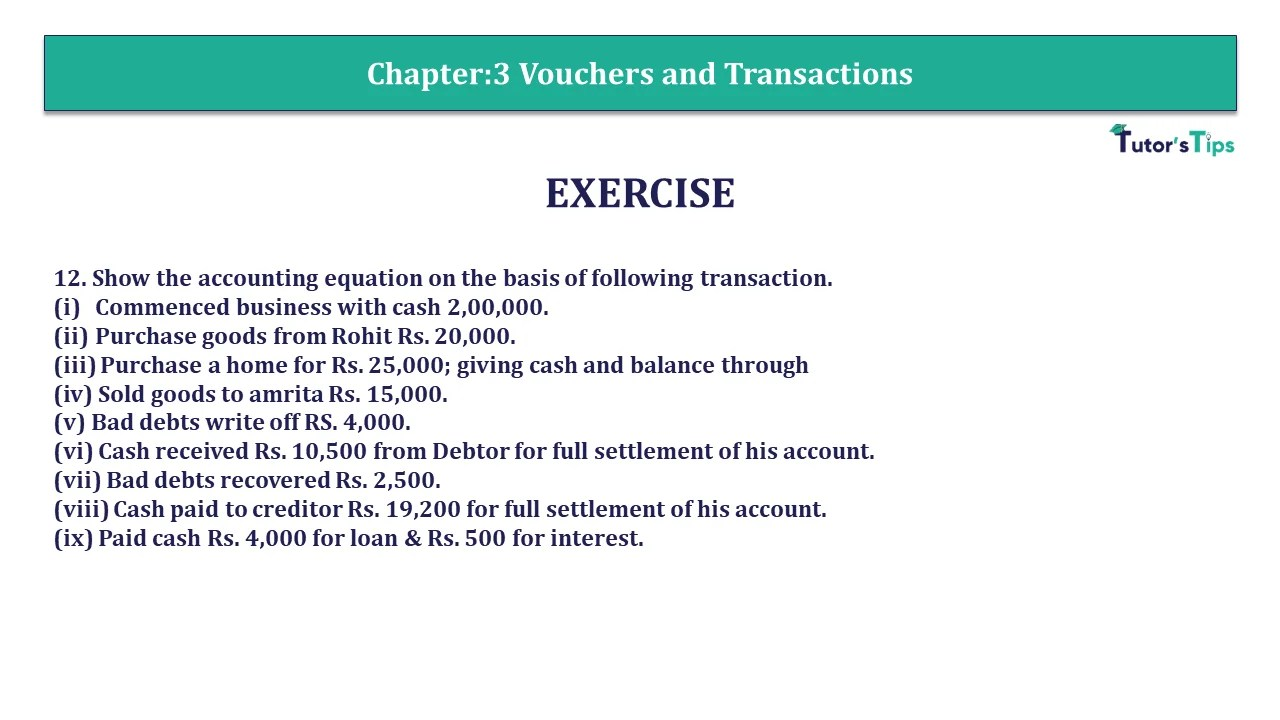Question 12 Chapter 3 - Unimax 12. Show the accounting equation on the basis of following transaction.(i)  Commenced business with ...Question 13 Chapter 3 - Unimax 13. Prepare accounting equation on the basis of following transaction. (i) Anant started business ...Question 14 Chapter 3 - Unimax 14. Show the effect of following transactions on Accounting equation. George started business with ...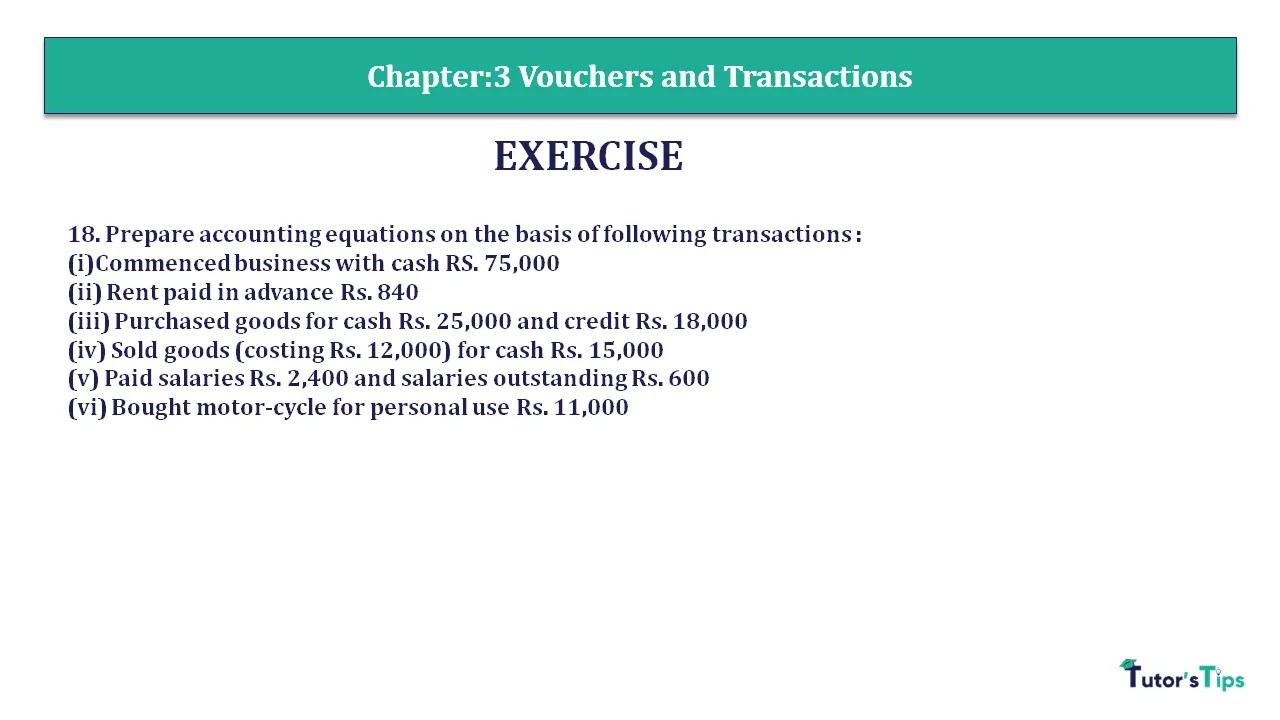18. Prepare accounting equations on the basis of following transactions :(i)Commenced business with cash RS. 75,000(ii) Rent paid in advance ...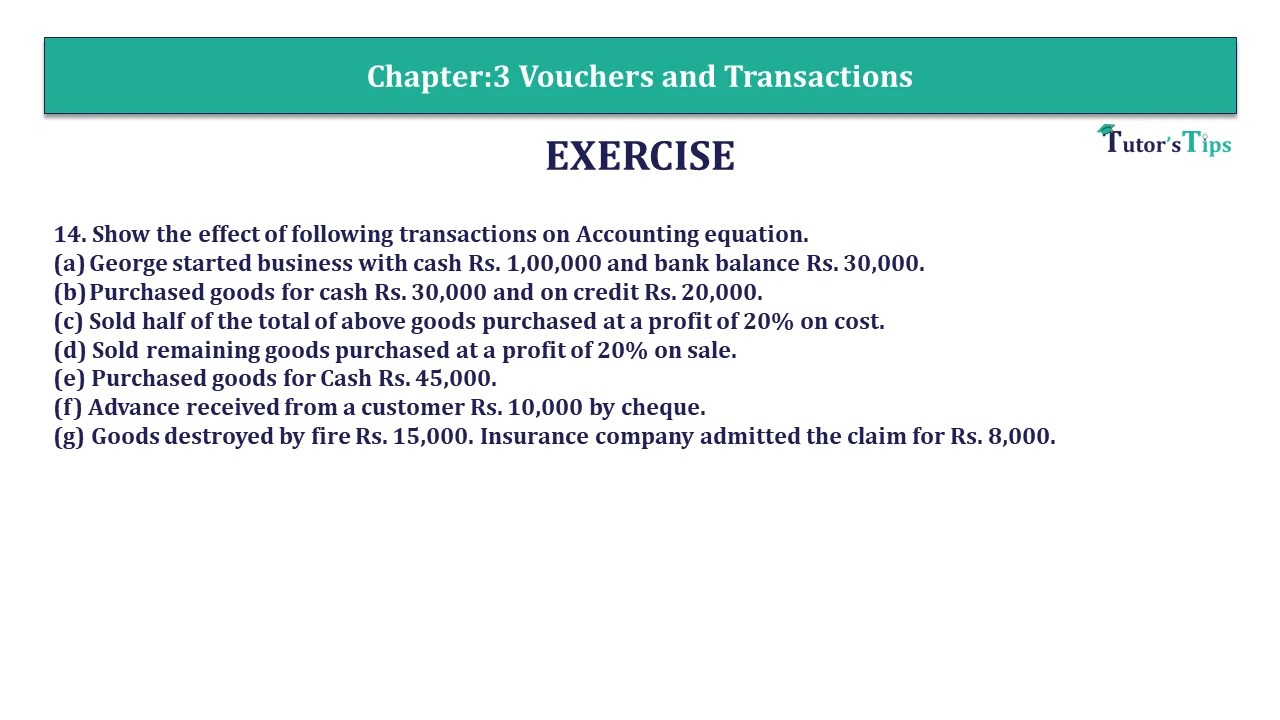Question 14 Chapter 3 - Unimax 14. Show the effect of following transactions on Accounting equation. (a) George started business ...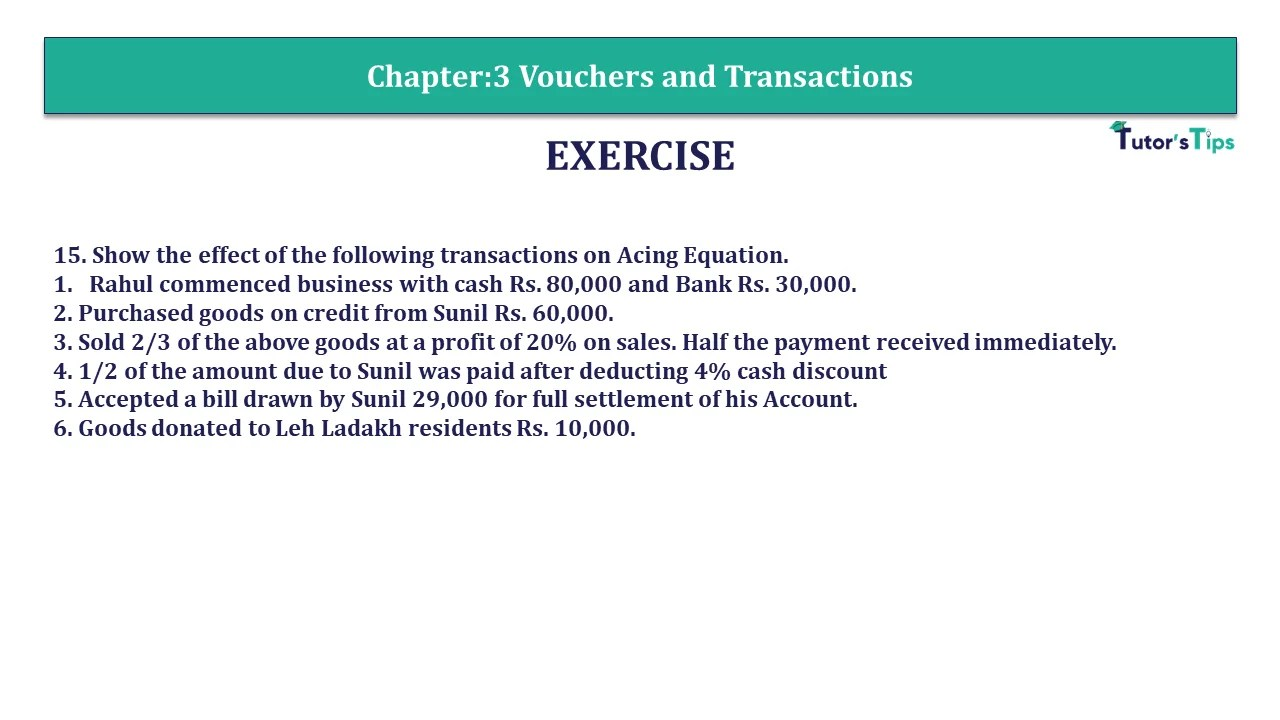Question 15 Chapter 3 - Unimax 15. Show the effect of the following transactions on acing Equation. 1) Rahul commenced ...Question 16 Chapter 3 - Unimax 16 Prepare the accounting action from the following:(i)  Started business with cash Rs. 6,00,000.(ii) ...Question 18 Chapter 3 - Unimax 18. Prepare accounting equations on the basis of following transactions :(i)Commenced business with cash ...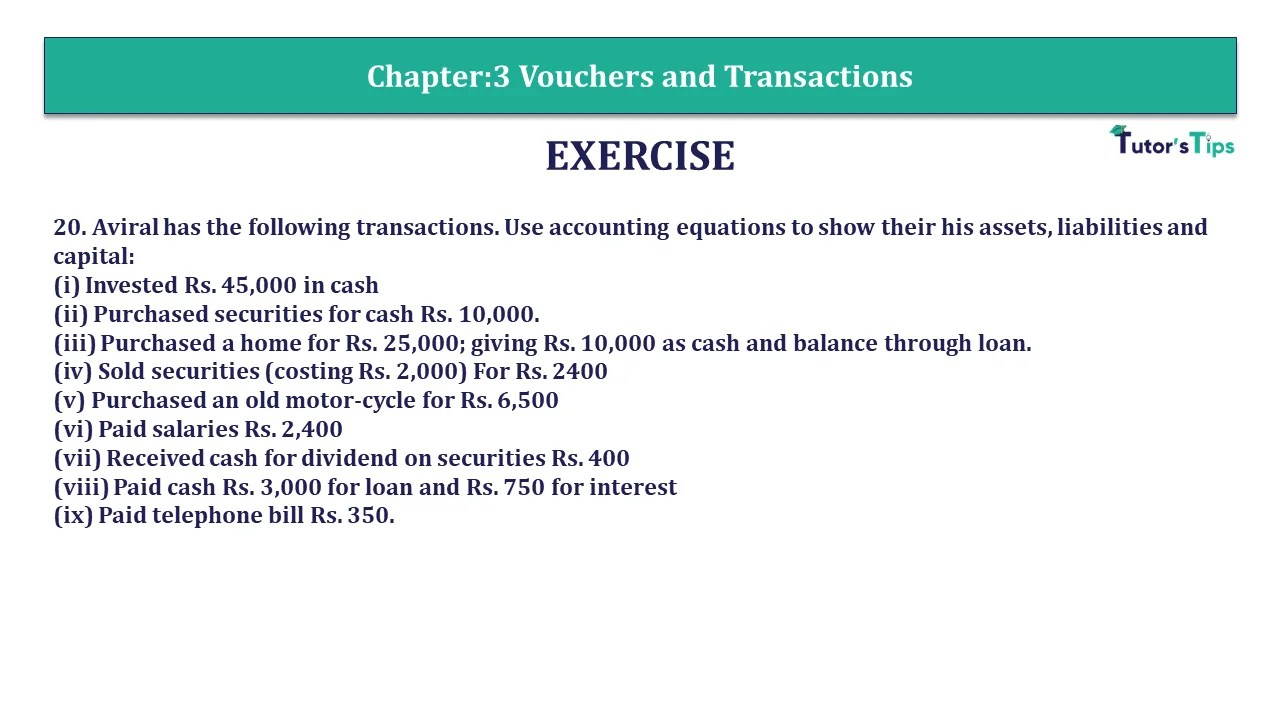Question 20 Chapter 3 - Unimax 20. Aviral has the following transactions. Use accounting equations to show their his assets, ...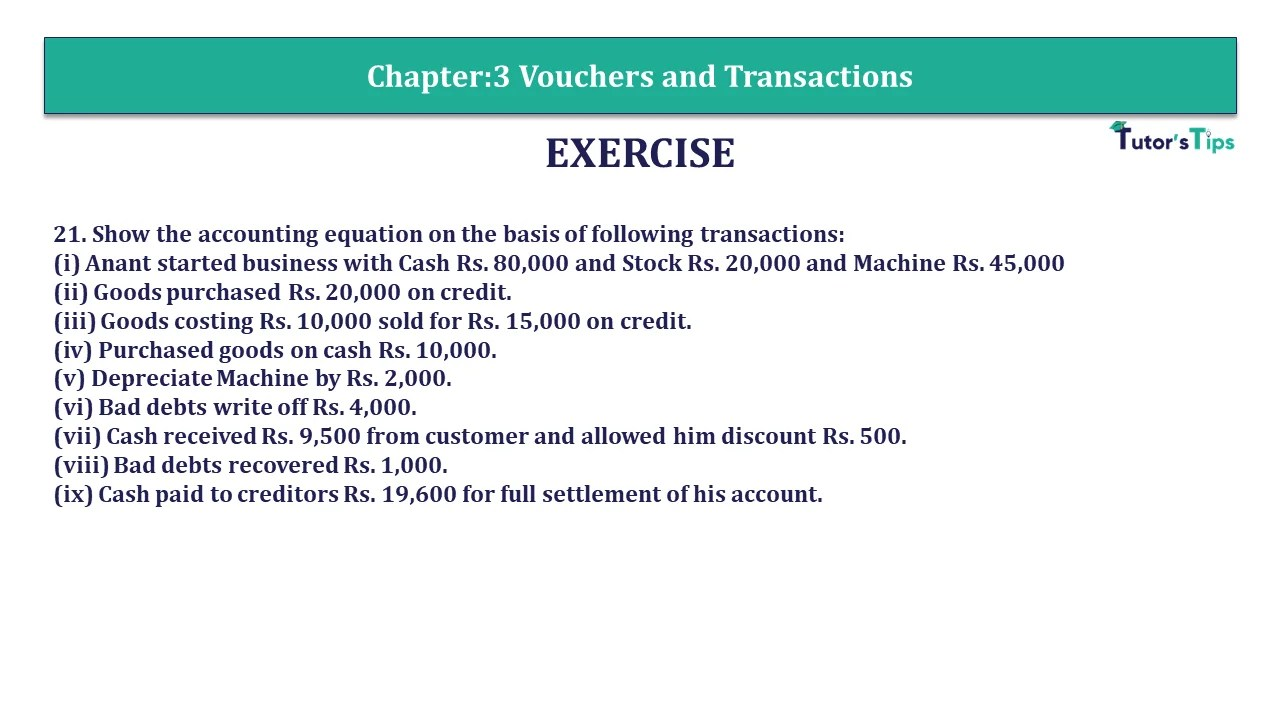Question 21 Chapter 3 - Unimax 21. Show the accounting equation on the basis of following transactions:(i) Anant started business ...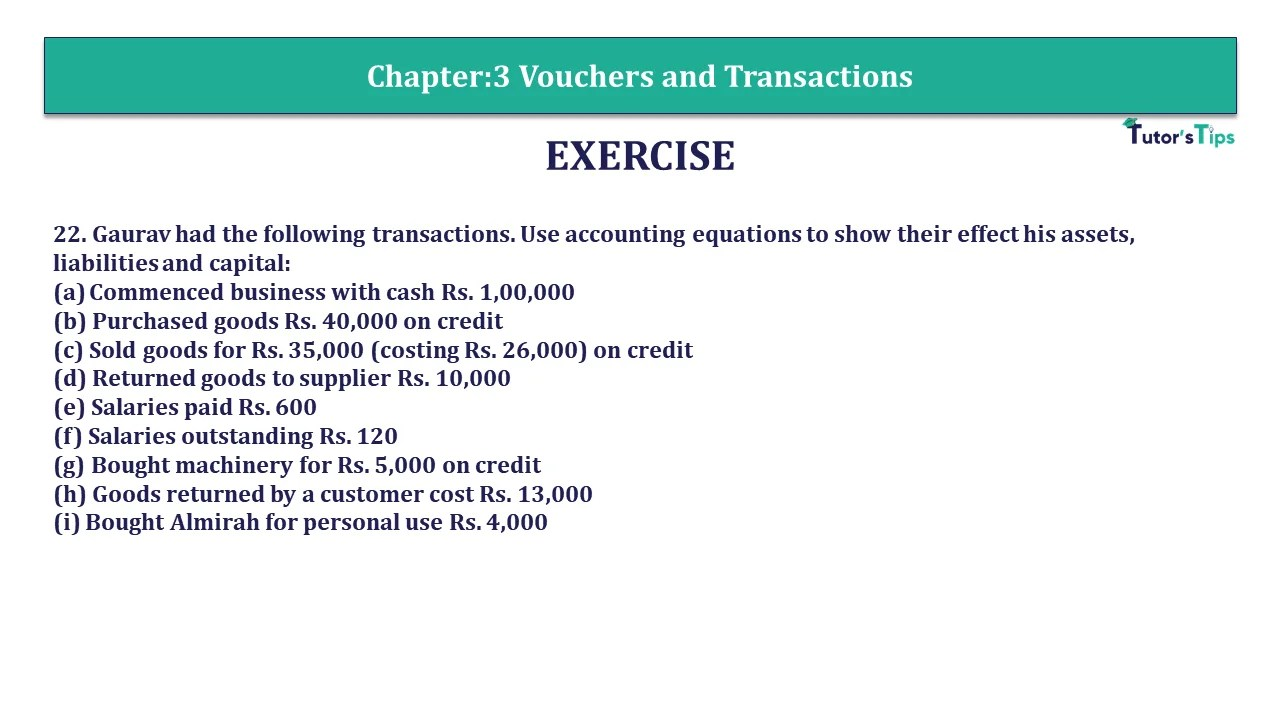Question 22 Chapter 3 - Unimax 22. Gaurav had the following transactions. Use accounting equations to show their effect his ...Question 23 Chapter 3 - Unimax 23. Prepare the Accounting Equation on the basis of the following: Rs. 1. Sahib ...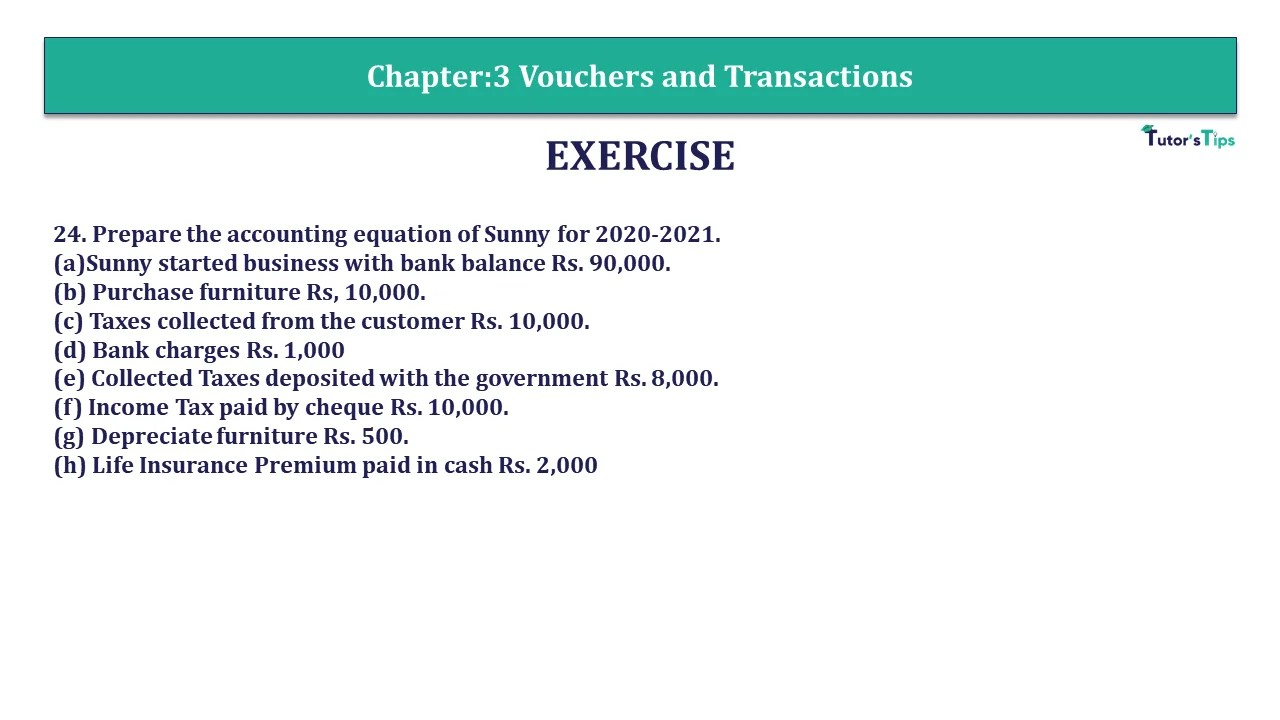Question 24 Chapter 3 - Unimax 24. Prepare the accounting equation of Sunny for 2020-2021.(a)Sunny started business with bank balance ...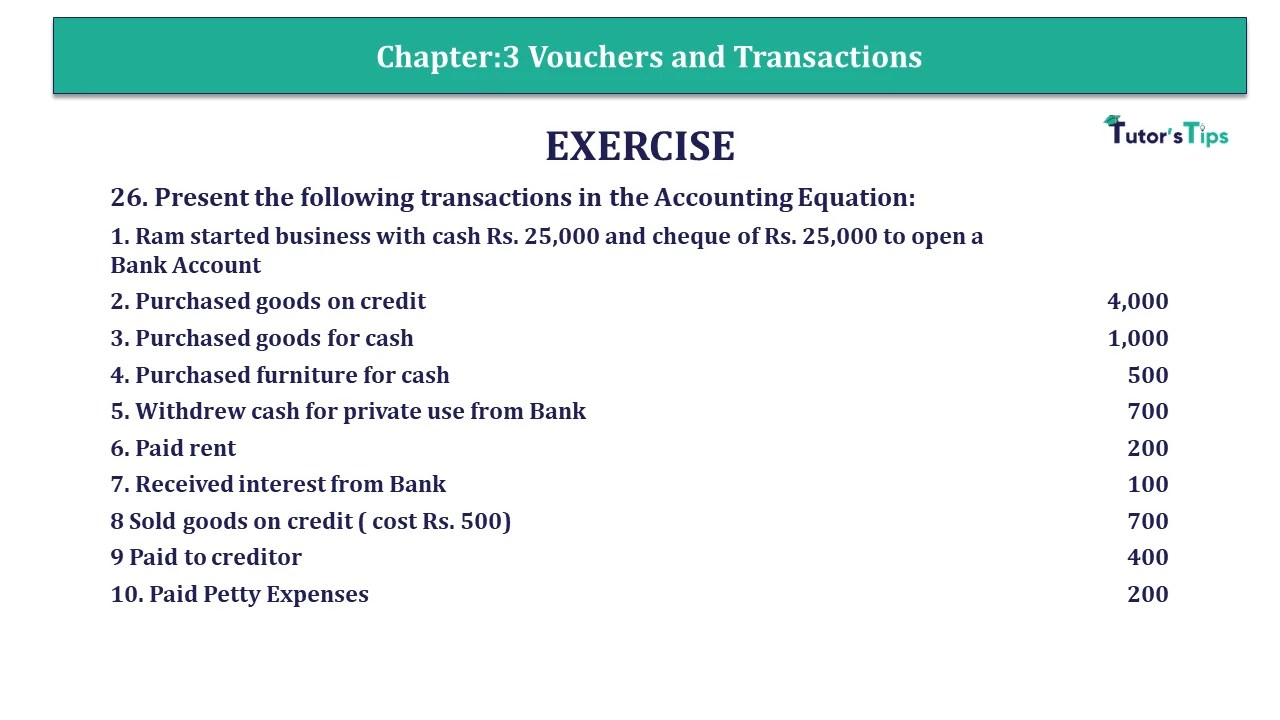Question 26 Chapter 3 - Unimax 26. Present the following transactions in the Accounting Equation: 1. Ram started business with ...